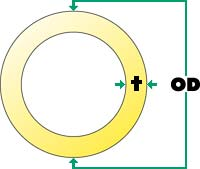# McCalc® Fusion Pressure Calculator

Use McElroy's fusion pressure calculator to quickly find the right fusion pressure for your job. To properly fuse pipe the fusion pressure must be adjusted so the pipe manufacturers recommended interfacial pressure is achieved.

We have several convenient ways to calculate fusion pressure. You can use our online tool below, download the Fusion Pressure Calculator App for your mobile device, or order a sliding calculator.

Wondering how it all works? Learn how to calculate fusion pressure.

## McCalc Mobile App

Always have a fusion pressure calculator with you no matter where you are.## Learn To Calculate Fusion Pressure

### Example

Using a 28 Machine from Chart A (at right) we will determine our gauge pressure.

Pipe Size = 8" IPS
OD of Pipe = 8.625
DR of Pipe = 11
IFP = 75 PSI
Measured Drag = 30 PSI

### Variable DefinitionsOD = Outside diameter
T = Wall thickness
PI = 3.1416
DR = Dimensional ratio
IFP = Manufacturers recommended interfacial pressure
TEPA = Total effective piston area
DRAG = Force required to move pipe

### Total effective piston area

Model High Force Standard Medium Force High Velocity Low Force Extra High Velocity
28 4.71 NA 1.67
412 11.78 6.01 3.14
618 11.78 6.01 3.14
500 NA 6.01 NA
824 29.44 15.32 9.45
1236 29.44 15.32 9.45
900 NA 15.32 NA
1648 31.42 14.14 NA
2065 31.42 NA NA

## Step 1. - Calculating Wall Thickness:

To determine wall thickness we will be using the Example (above).

 T = OD DR = Wall thickness

Now the Example and the formula above we will calculate the wall thickness.

 T = 8.625 11 = .784

## Step 2. - Finding Total Effective Piston Area

Use Chart A (above) to find the TEPA of your machine.

TEPA = 4.710 (we are using the 28 as an example)

## Step 3. - Calculating Gauge Pressure

Using the information we have gathered from above.

 (OD - T) x T x PI x IFP TEPA + DRAG = Gauge Pressure

## Step 4. - Completed Formula

Now using all the information and the formula above we will find the gauge pressure.

 (8.625 - .784) x .784 x 3.1416 x 75 4.710 + 30 PSI = 338 PSI